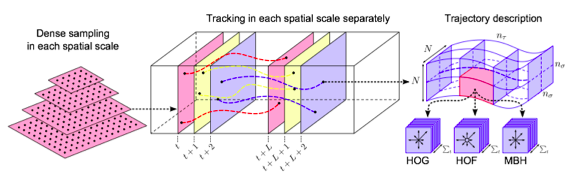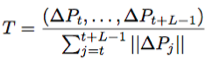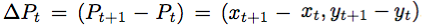### BACI Simple Install and Compile Guide

This is a guideline for a course of this semester, for which I am serving as a teaching assistant. I publish it here in hope that it can also help someone else who want to use BACI.

### Dense Trajectory Notes

Notes of Dense Trajectories• densely sample feature points in each frame
• track points in the video based on optical flow.
• compute multiple descriptors along the trajectories of feature points to capture shape, appearance and motion information.
• ### Dense Sampling

• Sampling step size $$W=5$$ pixels
• # spatial scales ≤ 8
• Spatial scale increase: $$1 / \sqrt{2}$$
• Removing points in homogeneous areas: $$T=0.001 \times \max_{i \in l}\min(\lambda_{i}^{1},\lambda_{i}^{2})$$, where $$(\lambda_{i}^{1},\lambda_{i}^{2})$$ are eigenvalues of point $$i$$ in image $$I$$ (the auto-correlation matrix).
• ### Descriptors

• Trajectory shape descriptor(TR):where L is the length of trajectory, and the displacement vectors• HOG – static appearance information
• HOF – local motion information
• MBH – motion descriptor for trajectories
• ### Format of DTF features

#### The format of the computed features

The features are computed one by one, and each one in a single line, with the following format:

frameNum mean_x mean_y var_x var_y length scale x_pos y_pos t_pos Trajectory HOG HOF MBHx MBHy

The first 10 elements are information about the trajectory:

• frameNum:     The trajectory ends on which frame
• mean_x:       The mean value of the x coordinates of the trajectory
• mean_y:       The mean value of the y coordinates of the trajectory
• var_x:        The variance of the x coordinates of the trajectory
• var_y:        The variance of the y coordinates of the trajectory
• length:       The length of the trajectory
• scale:        The trajectory is computed on which scale
• x_pos:        The normalized x position w.r.t. the video (0~0.999), for spatio-temporal pyramid
• y_pos:        The normalized y position w.r.t. the video (0~0.999), for spatio-temporal pyramid
• t_pos:        The normalized t position w.r.t. the video (0~0.999), for spatio-temporal pyramid

The following element are five descriptors concatenated one by one:

• Trajectory:    2x[trajectory length] (default 30 dimension)
• HOG:           8x[spatial cells]x[spatial cells]x[temporal cells] (default 96 dimension)
• HOF:           9x[spatial cells]x[spatial cells]x[temporal cells] (default 108 dimension)
• MBHx:          8x[spatial cells]x[spatial cells]x[temporal cells] (default 96 dimension)
• MBHy:          8x[spatial cells]x[spatial cells]x[temporal cells] (default 96 dimension)
1. ### Improved Dense Trajectories

• Explicit camera motion estimation
• Assumption: two consecutive frames are related by a homography.
• Match feature points between frames using SURF descriptors and dense optical flow
• Removing inconsistent matches due to humans: use a human detector to remove matches from human regions (computation expensive)
• Estimate a homography with RANSAC with these matches

### References:

1. H Wang, C Schmid, Action recognition with improved trajectories, ICCV 2013
2. H Wang, A Kläser, C Schmid, CL Liu, Dense trajectories and motion boundary descriptors for action recognition, International Journal of Computer Vision, May 2013, Volume 103, Issue 1, pp 60-79

### Parallelize mex file in Matlab

By default mex file cannot be executed in parallel in Matlab, which will be a major bottleneck for Matlab program. Fortunately, there is a way to execute loop operations in parallel -- parfor. Matlab provides a good tutorial on parfor: Getting Started with parfor.

One tricky problem with parfor is dealing with reduction assignments, which means the value of some variables are updated by each iteration, such as assignment to an array/matrix indexed by loop index.  My suggestion is to separate the loop into two parts: one part deal with non-reduction assignment with parfor, and the other part do the reduction assignment with traditional for loop.

If you cannot save all the parfor-assigned variables in memory, then you must save them into mat file and load them in the next for-loop. In the parfor-loop, command "save" cannot  be used directly because it violates the transparency(God knows what does it mean!). As an alternative, you can create a wrapper function of save and call that function instead. FOr example:

Save the following as "parsave.m":
function parsave(fname, x,y) save(fname, 'x', 'y') end 
Then run it with:
parfor ii = 1:4 x = rand(10,10); y = ones(1,3); parsave(sprintf('output%d.mat', ii), x, y); end

References:

1. parfor manual: http://www.mathworks.com/help/distcomp/parfor.html
2. Getting Started with parfor: http://www.mathworks.com/help/distcomp/getting-started-with-parfor.html
3. How do I use SAVE with a PARFOR loop: http://www.mathworks.com/support/solutions/en/data/1-D8103H/?product=DM&solution=1-D8103H

### Notes of Advanced Machine Learning(I)

1. Two different ways represent a distribution over several random variables: (1) product of conditional probabilities: $$p(x_1,x_2,x_3,x_4)=p(x_4)p(x_3|x_4)p(x_2|x_3,x_4)p(x_1|x_2,x_3,x_4)$$ and (2) global energy function: $$p(x_1,x_2,x_3,x_4)=\frac{1}{Z}e^{-E(x_1,x_2,x_3,x_4)}$$, where $$Z$$ is the partition function.
2. Directed graphical models use conditional probabilities, which undirected graphical models use energy functions that are a sum of several terms. Deep belief net(DBN) is a hybrid model.
1. ### Probabilistic Model

Two different ways represent a distribution over several random variables:

• product of conditional probabilities: p(x1,x2,x3,x4)=p(x4)p(x3|x4)p(x2|x3,x4)p(x1|x2,x3,x4)

• global energy function:

p(x1,x2,x3,x4)=1Ze{-E(x1,x2,x3,x4)},

where Zis the partition function.

Directed graphical models use conditional probabilities(Bayesian networks), while undirected graphical models(Markov random fields, Boltzmann machines) use energy functions that are a sum of several terms. Deep belief net(DBN) is a hybrid model.

#### Directed Graphs

Directed graphs are useful for expressing causal relationships between random variables.

• The joint distribution defined by the graph is given by the product of a conditional distribution for each node conditioned on its parents.

• For example, the joint distribution over x1,,x7 factorizes:

p(x)=p(x1)p(x2)p(x3)p(x4|x1,x2,x3)p(x5|x1,x3)p(x6|x4)p(x7|x4,x5)

#### Markov Random Fields

p(x)=1Zcc(xc)

• Each potential function is a mapping from joint configurations of random variables in a clique to non-negative real numbers.

• The choice of potential functions is not restricted to having specific probabilistic interpretations.

• Potential functions are often represented as exponentials:

p(x)=1Zcc(xc)=1Z(-cE(xc))=1Z(-E(x)) (Boltzmann distribution)

• Computing Z is very hard, which represents a major limitation of undirected models.

1. ### Singular Value Decomposition

Singular Value Decomposition(SVD) is a factorization of a real or complex matrix. Formally, the singular value decomposition of an mn matrix M is a factorization of the form

M=UV*

where U is a mm unitary matrix,  is an mn rectangular diagonal matrix with nonnegative real numbers on the diagonal, and V*(the conjugate transpose of V: (V*)ij=Vji, for real matrix, it equals the transpose) is an nn unitary matrix.

A complex square matrix U is unitary if U*U=UU*=I.

The diagonal entries ij of  are known as the singular values of M, which means they are the square roots of the eigenvalues of matrix MM*. The m columns of U and n columns of V are called the left-singular vectors and right-singular vectors of M, respectively.

The SVD and the eigendecomposition are closely related:

• The left-singular vectors of M(columns of U) are eigenvectors of MM*.

• The right-singular vectors of M(columns of V) are eigenvectors of M*M.

• The non-zero singular values of M(diagonal entries of ) are the square roots of the non-zero eigenvalues of both M*M and MM*.

References:

1. U Toronto CSC2535: http://www.cs.toronto.edu/~hinton/csc2535/lectures.html

### "undefined reference" error during installing OpenCV

Many people(including me) met the infamous "undefined reference" error during installing OpenCV, such as

../../lib/libopencv_highgui.so.2.4.1: undefined reference to avpriv_vorbis_parse_extradata’
../../lib/libopencv_highgui.so.2.4.1: undefined reference to av_des_init’
../../lib/libopencv_highgui.so.2.4.1: undefined reference to av_rc4_crypt’
../../lib/libopencv_highgui.so.2.4.1: undefined reference to av_aes_crypt’
../../lib/libopencv_highgui.so.2.4.1: undefined reference to av_des_mac’
../../lib/libopencv_highgui.so.2.4.1: undefined reference to av_tree_destroy’
../../lib/libopencv_highgui.so.2.4.1: undefined reference to av_sha_update’
collect2: ld returned 1 exit status
make: *** [bin/opencv_perf_imgproc] Error 1
make: *** [modules/imgproc/CMakeFiles/opencv_perf_imgproc.dir/all] Error 2
make: *** [all] Error 2

This error was caused by lacking of or improper installation of ffmpeg. If you are sure the ffmpeg is successfully installed, and you can find "– FFMPEG: YES" after cmake opencv, then you'd better think about adding option "--enable-shared " when configure ffmpeg. After re-installing ffmpeg, then this "undefined reference" error should disappear.

PS: A very good guideline of installing OpenCV on Ubuntu:

# A Comprehensive Guide to Installing and Configuring OpenCV 2.4.2 on Ubuntu

PPS: Damn Ubuntu!!!

### Build Dense Trajectory Codes in Ubuntu

Even when the OpenCV and ffmpeg have been successfully installed, you still may meet the error of "undefined reference to main" when building the Dense Trajectory code. Since the author didn't provide a solution, I share my workaround here -- just use command g++ directly:

### Matlab Startup Script

You can create a script named startup.m under userpath(in Windows and Mac OSX, default path is Documents/MATLAB). You can add whatever Matlab commands you want into startup.m. For example, to add /usr/local/bin into PATH, you can add command

setenv('PATH', [getenv('PATH') ':/usr/local/bin']);

into startup.m.

BTW, every time Matlab starts, configure file \$MATLABROOT/toolbox/local/matlabrc.m will be executed.

### Counting Unique k-mers -- My First Go Program

Previously, I wrote a Perl program to count the number of unique k-mers. It is very convenient to implement it in Perl, because Perl supports hash-of-hashes(which could dynamically count distinct k-mers) and sorting hashes by value(which can easily list the occurrences of unique k-mers in descending order).

Unfortunately, comparing to C++, Perl program usually costs more memory and longer time. This is not a big issue when the FASTA files are not large(smaller than 400MB). However, when handling larger FASTA files, my Perl program would require more than 8GB memory, which exceeds the limits of my computer. Therefore, I decided to rewrite the program by Go, a system language that supports concurrent features and can has C/C++-comparable speed.

The syntax of Go is very different from C/C++, which takes me a whole day familiarize. Some operations that can be implemented easily in Perl can only be done in complicated way, such as calling system command, reading file line-by-line, writing file and sorting map by value. Since this small tool doesn't involve network and concurrency, I cannot compare the difficulty of writing Go program to implement such work.

Although the experience of the first Go program is not happy, I still think it is necessary to continue learning Go language, because Go program really runs very fast and saves memory(comparing to Perl script). And you don't need to worry about the tricky part of C++.

### Linear Space Sequence Alignment

For explanation to linear space sequence alignment, please refer to http://ai.stanford.edu/~serafim/CS262_2007/notes/lecture3.pdf.

The algorithm and equation I used was from the textbook Algorithm Design by Jon Kleinberg and Éva Tardos(2005):

### Implementation of Strassen's Algorithm for Matrix Multiplication

Strassen's algorithm is not the most efficient algorithm for matrix multiplication, but it was the first algorithm that was theoretically faster than the naive algorithm. There is very good explanation and implementation of Strassen's algorithm on Wikipedia.

However, the implementation of Strassen's algorithm cannot be used directly, because it  just sets the base case of the divide-and-conquer to be 1x1 matrix, which would consume huge time cost for iteration. If set the base case to 2x2 matrix, which means 2x2 matrix and 1x1 matrix will be multiplied by naive algorithm, then the Strassen's algorithm will be more efficient for matrices larger than 512x512.When set base case to 2x2 matrix, then the Strassen's algorithm will surpass naive algorithm for matrices larger than 512x512.When set base case to 6x6 matrix, then the Strassen's algorithm will surpass naive algorithm for matrices larger than 128x128.


/*------------------------------------------------------------------------------*/
// matrix_mult.cc -- Implementation of matrix multiplication with
// Strassen's algorithm.
//
// Compile this file with gcc command:
// g++ -Wall -o matrix_mult matrix_mult.cc

#include <stdio.h>
#include <stdlib.h>
#include <time.h>
#include <ctype.h>
#include <unistd.h>
#include <iostream>
#include <fstream>
#include <cmath>
#include <cstring>

using namespace std;

// This function allocates the matrix
inline double** allocate_matrix(int n)
{
double** mat=new double*[n];
for(int i=0;i<n;++i)
{
mat[i]=new double[n];
memset(mat[i],0,sizeof(double)*n);
}

return (mat); // returns the pointer to the vector.
}

/*------------------------------------------------------------------------------*/
// This function unallocates the matrix (frees memory)
inline void free_matrix(double **M, int n)
{
for (int i = 0; i < n; i++)
{
delete [] M[i];
}

delete [] M; // frees the pointer /
M = NULL;
}

/*------------------------------------------------------------------------------*/
// function to sum two matrices
inline void sum(double **a, double **b, double **result, int tam) {

int i, j;

for (i = 0; i < tam; i++) {
for (j = 0; j < tam; j++) {
result[i][j] = a[i][j] + b[i][j];
}
}
}

/*------------------------------------------------------------------------------*/
// function to subtract two matrices
inline void subtract(double **a, double **b, double **result, int tam) {

int i, j;

for (i = 0; i < tam; i++) {
for (j = 0; j < tam; j++) {
result[i][j] = a[i][j] - b[i][j];
}
}
}

/*------------------------------------------------------------------------------*/
// naive method
void naive(double** A, double** B,double** C, int n)
{
for (int i=0;i<n;i++)
for (int j=0;j<n;j++)
for(int k=0;k<n;k++)
C[i][j] += A[i][k]*B[k][j];
}

/*------------------------------------------------------------------------------*/
// Strassen's method
void strassen(double **a, double **b, double **c, int tam)
{
// Key observation: call naive method for matrices smaller than 2 x 2
if(tam <= 4)
{
naive(a,b,c,tam);
return;
}

// other cases are treated here:
int newTam = tam/2;
double **a11, **a12, **a21, **a22;
double **b11, **b12, **b21, **b22;
double **c11, **c12, **c21, **c22;
double **p1, **p2, **p3, **p4, **p5, **p6, **p7;

// memory allocation:
a11 = allocate_matrix(newTam);
a12 = allocate_matrix(newTam);
a21 = allocate_matrix(newTam);
a22 = allocate_matrix(newTam);

b11 = allocate_matrix(newTam);
b12 = allocate_matrix(newTam);
b21 = allocate_matrix(newTam);
b22 = allocate_matrix(newTam);

c11 = allocate_matrix(newTam);
c12 = allocate_matrix(newTam);
c21 = allocate_matrix(newTam);
c22 = allocate_matrix(newTam);

p1 = allocate_matrix(newTam);
p2 = allocate_matrix(newTam);
p3 = allocate_matrix(newTam);
p4 = allocate_matrix(newTam);
p5 = allocate_matrix(newTam);
p6 = allocate_matrix(newTam);
p7 = allocate_matrix(newTam);

double **aResult = allocate_matrix(newTam);
double **bResult = allocate_matrix(newTam);

//dividing the matrices in 4 sub-matrices:
for (int i = 0; i < newTam; i++) {
for (int j = 0; j < newTam; j++) {
a11[i][j] = a[i][j];
a12[i][j] = a[i][j + newTam];
a21[i][j] = a[i + newTam][j];
a22[i][j] = a[i + newTam][j + newTam];

b11[i][j] = b[i][j];
b12[i][j] = b[i][j + newTam];
b21[i][j] = b[i + newTam][j];
b22[i][j] = b[i + newTam][j + newTam];
}
}

// Calculating p1 to p7:

sum(a11, a22, aResult, newTam); // a11 + a22
sum(b11, b22, bResult, newTam); // b11 + b22
strassen(aResult, bResult, p1, newTam); // p1 = (a11+a22) * (b11+b22)

sum(a21, a22, aResult, newTam); // a21 + a22
strassen(aResult, b11, p2, newTam); // p2 = (a21+a22) * (b11)

subtract(b12, b22, bResult, newTam); // b12 - b22
strassen(a11, bResult, p3, newTam); // p3 = (a11) * (b12 - b22)

subtract(b21, b11, bResult, newTam); // b21 - b11
strassen(a22, bResult, p4, newTam); // p4 = (a22) * (b21 - b11)

sum(a11, a12, aResult, newTam); // a11 + a12
strassen(aResult, b22, p5, newTam); // p5 = (a11+a12) * (b22)

subtract(a21, a11, aResult, newTam); // a21 - a11
sum(b11, b12, bResult, newTam); // b11 + b12
strassen(aResult, bResult, p6, newTam); // p6 = (a21-a11) * (b11+b12)

subtract(a12, a22, aResult, newTam); // a12 - a22
sum(b21, b22, bResult, newTam); // b21 + b22
strassen(aResult, bResult, p7, newTam); // p7 = (a12-a22) * (b21+b22)

// calculating c21, c21, c11 e c22:

sum(p3, p5, c12, newTam); // c12 = p3 + p5
sum(p2, p4, c21, newTam); // c21 = p2 + p4

sum(p1, p4, aResult, newTam); // p1 + p4
sum(aResult, p7, bResult, newTam); // p1 + p4 + p7
subtract(bResult, p5, c11, newTam); // c11 = p1 + p4 - p5 + p7

sum(p1, p3, aResult, newTam); // p1 + p3
sum(aResult, p6, bResult, newTam); // p1 + p3 + p6
subtract(bResult, p2, c22, newTam); // c22 = p1 + p3 - p2 + p6

// Grouping the results obtained in a single matrix:
for (int i = 0; i < newTam ; i++) {
for (int j = 0 ; j < newTam ; j++) {
c[i][j] = c11[i][j];
c[i][j + newTam] = c12[i][j];
c[i + newTam][j] = c21[i][j];
c[i + newTam][j + newTam] = c22[i][j];
}
}

// deallocating memory (free):
free_matrix(a11, newTam);
free_matrix(a12, newTam);
free_matrix(a21, newTam);
free_matrix(a22, newTam);

free_matrix(b11, newTam);
free_matrix(b12, newTam);
free_matrix(b21, newTam);
free_matrix(b22, newTam);

free_matrix(c11, newTam);
free_matrix(c12, newTam);
free_matrix(c21, newTam);
free_matrix(c22, newTam);

free_matrix(p1, newTam);
free_matrix(p2, newTam);
free_matrix(p3, newTam);
free_matrix(p4, newTam);
free_matrix(p5, newTam);
free_matrix(p6, newTam);
free_matrix(p7, newTam);
free_matrix(aResult, newTam);
free_matrix(bResult, newTam);

} // end of Strassen function

/*------------------------------------------------------------------------------*/
// Generate random matrices
void gen_matrix(double** M,int n)
{
for(int i=0;i<n;++i)
{
for(int j=0;j<n;++j)
{
M[i][j]=rand()%100;
//M[i][j]=1;
}
}
}

/*------------------------------------------------------------------------------*/
// print matrix M using specied fstream
void print_matrix(fstream& fs, double** M, int n)
{
for(int i=0;i<n;++i)
{
for(int j=0;j<n;++j)
{
fs<<M[i][j]<<" ";
}
fs<<endl;
}
fs<<endl;
}

/*------------------------------------------------------------------------------*/
// record the generated matrix and the final product
void mat_mult_log(double** A, double** B,double** C,int n,char* file)
{
fstream fs;
fs.open(file,fstream::out);

fs<<"Random Matrix A:"<<endl;
print_matrix(fs,A,n);
fs<<"Random Matrix B:"<<endl;
print_matrix(fs,B,n);
fs<<"C=A * B"<<endl;
print_matrix(fs,C,n);

fs.close();
}

/*------------------------------------------------------------------------------*/

int main(int argc, char** argv)
{
srand(time(NULL));

int mdim=2; // matrix dimension
char* output=NULL;
bool is_strassen=false;
int c;

while ((c = getopt (argc, argv, "sn:o:")) != -1)
{
switch (c)
{
case 's':
is_strassen=true;
break;
case 'n':
mdim = pow((int)2,atoi(optarg)); // 2^n dimensions
break;
case 'o':
output = optarg; // 2^n dimensions
break;
case '?':
if (optopt == 'n')
fprintf (stderr, "Option -%c requires an argument.\n", optopt);
else if (isprint (optopt))
fprintf (stderr, "Unknown option -%c'.\n", optopt);
else
fprintf (stderr,
"Unknown option character \x%x'.\n",
optopt);
return 1;
default:
abort ();
}
}

// create new matrices
double** A=allocate_matrix(mdim);
double** B=allocate_matrix(mdim);
double** C=allocate_matrix(mdim);
gen_matrix(A,mdim);
gen_matrix(B,mdim);

// matrices multiplication
if(is_strassen)
strassen(A,B,C,mdim);
else
naive(A,B,C,mdim);

if(output!=NULL)
mat_mult_log(A,B,C,mdim,output);

free_matrix(A,mdim);
free_matrix(B,mdim);
free_matrix(C,mdim);

return 0;
}`